# First-order differential equation

## Definition

### Formal description

The term first-order differential equation is used for any differential equation whose order is 1. In other words, it is a differential equation of the form: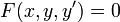$F(x,y,y') = 0$

where$F$ is an expression (function) involving three variables. Note that$F$ must make use of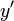$y'$ (also written as$dy/dx$), but it could ignore$x$ or$y$.

The theory and terminology follows that for the general concept of differential equation.

### Solution concept

• Functional solution: A function$f$ on the domain of interest is said to be a solution (or functional solution) to the equation if, when we plug in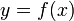$y = f(x)$, the equation holds true for all$x$ in the domain, i.e.:$F(x,f(x),f'(x)) = 0 \ \forall \ x \in \operatorname{dom}(f)$

Note that in cases of functions defined on closed intervals, we exclude checking the conditions on the boundary of the domain because two-sided derivatives don't make sense at the boundary.

• Relational solution: A relation$R(x,y) = 0$ is termed a relational solution to the equation if$F(x,y,y') = 0$ holds true for all$x,y$ if we calculate the derivative$y'$ using implicit differentiation.

### Initial value problem

An initial value problem is a first-order differential equation$F(x,y,y') = 0$

along with a point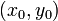$(x_0,y_0)$.

A functional solution to the initial value problem is a solution$y = f(x)$ to the first-order differential equation such that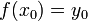$f(x_0) = y_0$.

A relational solution to the initial value problem is a solution$R(x,y) = 0$ to the first-order differential equation such that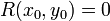$R(x_0,y_0) = 0$.

## Terminology

### Solution terminology

Term Meaning Example
particular solution a function or relation that is a solution for the equation (see #Solution concept). A solution in the form of a function$y = f(x)$ is termed a functional solution and a solution in the form of a relation$R(x,y) = 0$ is termed a relational solution.$y = \sin x$ is a functional solution to$y^2 + y'^2 = 1$.
solution family a family of functions or relations, with one or more parameters possibly subject to some constraints, such that for every choice of parameter values subject to those constraints, we get a particular solution.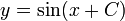$y = \sin(x + C)$ with parameter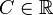$C \in \R$, is a solution family for$y^2 + y'^2 = 1$.
general solution a solution family that covers all solutions (or almost all solutions, possibly excluding some exceptions) The general solution to$y' = 0$ is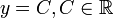$y = C, C \in \R$.
solution to initial value problem a particular solution that satisfies the initial value condition. A particular solution to$y + y' = 0$ satisfying$y(0) = 1$ is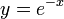$y = e^{-x}$.

## Facts

• As a general principle, the way to solve a first-order differential equation is to convert it to an integration problem. The additive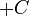$+C$ appearing in the indefinite integration gives the freely varying parameter for the solution family.
• As a general principle, the number of degrees of freedom (i.e., the number of independent freely varying parameters) in the general solution to a first-order differential equation is 1. In other words, we expect the solution space to be one-dimensional.
• As a general principle, the number of solutions to an initial value problem should be finite. If the differential equation is nice enough, then there should be a unique solution to any initial value problem.

## Geometric description of solutions

This geometric description is qualitatively different for first-order differential equations compared to higher-order differential equations.

A solution curve to a differential equation is a curve in the$xy$-plane corresponding to any solution to the differential equation. For instance, if$R(x,y) = 0$ is a relational solution, then the curve$R(x,y) = 0$ gives a solution curve for the differential equation.

For first-order differential equations, we generally expect that specifying a point on the curve uniquely determines the solution curve (because that's an initial value specification). Thus, the solution curves are expected to be (mostly) non-intersecting curves that (hopefully) cover the plane or a large part of the plane. This general expectation is likely to be met for first-order first-degree differential equations. For first-order differential equations of degree$n$, we generally expect up to$n$ solution curves through a generic point.

## Solution strategies

### Solution strategies in particular cases

Below are some formats of equations for which general strategies are known. Note that the letter$f$ is no longer used for the solution function but may be used for other functions.:

Equation type Degree (if polynomial in highest order derivative) Quick summary of solution strategy
first-order linear differential equation which in simplified form looks like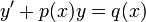$y' + p(x)y = q(x)$ 1 Use the integrating factor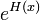$e^{H(x)}$ where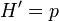$H'=p$. The general solution is$y = Ce^{-H(x)} + e^{-H(x)}\int p(x)e^{H(x)} \, dx$
separable differential equation which is of the form$y' = f(x)g(y)$ (any first-order first-degree autonomous differential equation is separable, though there are separable differential equations that aren't autonomous) 1 Separate and solve as$\int \frac{dy}{g(y)} = \int f(x) \, dx$. Also find solutions corresponding to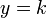$y = k$ where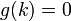$g(k) = 0$.
first-order exact differential equation$F(x,y,y') = 0$ 1 Try to find a relation$R(x,y)$ such that$F(x,y,y') = \frac{d}{dx}[R(x,y)]$ using implicit differentiation. Finding the$R$, even if it does exist, can be tricky.
Bernoulli differential equation$y' + p(x)y = q(x)y^n$ (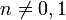$n \ne 0,1$) 1 Divide both sides by$y^n$ (set aside possible stationary solution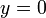$y = 0$), then substitute$w = 1/y^{n-1}$ to get a first-order linear differential equation with dependent variable$w$ and independent variable$x$.
Clairaut's equation which is of the form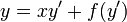$y = xy' + f(y')$ need not be polynomial; if polynomial, may have any degree$y = Cx + f(C)$ with$C \in \R$ (all straight lines) and a single other solution explicitly described as the solution to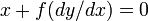$x + f(dy/dx) = 0$, given by$x = -f'(p), y = f(p) - pf'(p)$ as a parametric curve in terms of$p$.
Lagrange equation$y = f(y')x + g(y')$ which is linear in$x$ and$y$ but not necessarily in$y'$ need not be polynomial; if polynomial, may have any degree General solution is a family of curves, each described as a parametric curve with parameter the derivative$y'$ (which we denote by$p$). There may be some special straight line solutions of the form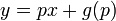$y = px + g(p)$ for values$p$ with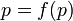$p = f(p)$.

### Reduction methods

The following are methods that may be used to reduce more complicated first-order differential equations to simpler ones:

Method Can it reduce the degree of the differential equation, and potentially convert a higher degree differential equation to first-degree differential equations? Description
substitution method for solving differential equations (the substitutions of interest are zeroth-order substitutions) No We put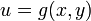$u = g(x,y)$ and replace one of the variables$x,y$ with$u$. Then, after solving, we plug back$u = g(x,y)$ to get the relational solutions between$x$ and$y$.
factorization method for solving differential equations Yes Bring everything to one side, factor it as a product of two expressions, then solve each as a separate differential equation, and combine solutions. Also, check for mixed solutions.